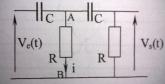# Determining the complex expression using Thevnin's theorem

I tried my best but I wasn't able to solve this can someone please provide me with a detailed solution.
Here 's the question : Establish the expression of Vs/Ve (complex) using Thevnin's theorem
Here is the circuit :I spent 4 hours trying to solve this but I had no clue how. I'am having problems summing the impedances and knowing where exactly to apply thevnin.A little guidance is appreciated. Thanks,

Thevenin’s Theorem states that it is possible to simplify any linear circuit, no matter how complex, to an equivalent circuit with just a single voltage source and series resistance connected to a load. More info here

So I started by giving an equivalent complex circuit since the courant is variable.
And as Thevnin's Th. states we need to remove the load, and then calculate the total impedance. Lets say the load is Vs(t) ( R ) now I need to sum Zc Zc Zr which are nor in parallel nor in series. I tried Kennelly's Transformation and things got even worse.

Last edited:

Hello Lilah,